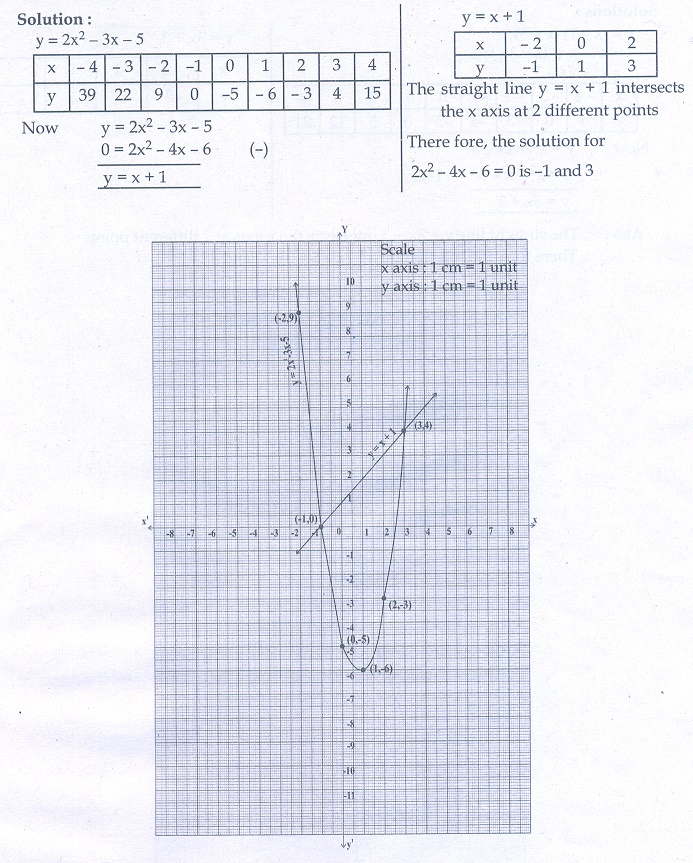Home | | Maths 10th Std | Exercise 3.15: Quadratic Graphs

## Chapter: 10th Mathematics : UNIT 3 : Algebra

Maths Book back answers and solution for Exercise questions - Graph the following quadratic equations and state their nature of solutions.

Exercise 3.15

1. Graph the following quadratic equations and state their nature of solutions.

(i) x2 − 9x + 20 = 0

(ii) x2 − 4x + 4 = 0

(iii) x2 + x + 7 = 0

(iv) x2 − 9 = 0

(v) x2 − 6x + 9 = 0

(vi) (2x − 3)(x + 2) = 02. Draw the graph of y = x2 −4 and hence solve x2 − x −12 = 03. Draw the graph of y = x2 + x and hence solve x2 + 1 = 04. Draw the graph of y = x2 + 3x + 2 and use it to solve x2 + 2x + 1 = 05. Draw the graph of y = x2 + 3x − 4  and hence use it to solve x2 + 3x − 4 = 06. Draw the graph of y = x2 − 5x − 6 and hence solve x2 − 5x − 14 = 07. Draw the graph of y = 2x2  − 3x − 5 and hence solve 2x2  − 4x − 6 = 08. Draw the graph of y = (x − 1)(x + 3) and hence solve x 2  − x − 6 = 01.(i) Real and unequal roots (ii) Real and equal roots (iii) No real roots

(iv) Real and unequal roots (v) Real and equal roots (vi) Real and unequal roots

2. –3, 4

3. No real roots

4. -1

5. -4 , 1

6. -2 ,7

7. –1, 3

8. –2, 3

Tags : Problem Questions with Answer, Solution | Mathematics , 10th Mathematics : UNIT 3 : Algebra
Study Material, Lecturing Notes, Assignment, Reference, Wiki description explanation, brief detail
10th Mathematics : UNIT 3 : Algebra : Exercise 3.15: Quadratic Graphs | Problem Questions with Answer, Solution | Mathematics